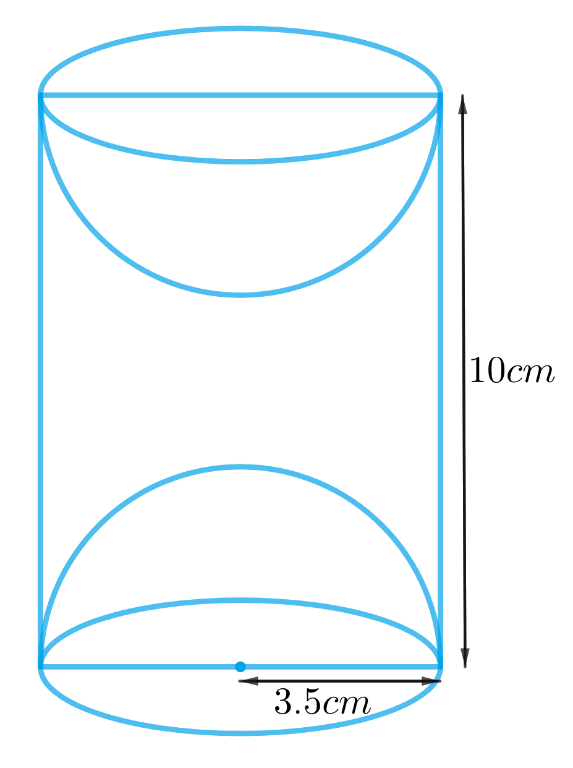# Ex.13.1 Q9 Surface Areas and Volumes Solution - NCERT Maths Class 10

## Question

A wooden article was made by scooping out a hemisphere from each end of a solid cylinder, as shown in Fig. 13.11. If the height of the cylinder is $$10 \rm{cm}$$, and its base is of radius $$3.5 \rm{cm,}$$find the total surface area of the article.Video Solution
Surface Areas And Volumes
Ex 13.1 | Question 9

## Text Solution

What is known?

The height of the cylinder is $$10 \rm{cm}$$, and radius of its base is $$3.5 \rm{cm}$$

What is unknown?

Total surface area of the article.

Reasoning:

From the figure it’s clear that radius of the hemispheres scooped out is same as the radius of base of the cylinder since both the hemispheres are of equal radius.
So, total surface area of the article only includes the CSA of both the hemispheres and the cylinder.

TSA of the article $$= 2 \times$$ CSA of the hemispherical part $$+$$ CSA of the cylindrical part

We will find the TSA of the article by using formulae;

CSA of the hemisphere  $$= 2\pi {r^2}$$

where r is the radius of the hemisphere

CSA of the cylinder  $$= 2\pi rh$$

where $$r$$ and $$h$$ are radius and height of the cylinder respectively.

Steps:

Height of the cylinder  $$= h = 10 \rm cm$$

Radius of the cylinder $$=$$ radius of the hemisphere $$= r = 3.5 \rm cm$$

TSA of the article $$=$$ $$2 \times$$  CSA of the hemispherical part $$+$$ CSA of the cylindrical part

\begin{align}&= 2 \times 2\pi {r^2} + 2\pi rh\\&= 2\pi r\left( {2r + h} \right)\\&= 2\! \times \!\!\frac{{22}}{7}\!\! \times \!3.5 \rm cm \!\times\! ( 2 \!\times\! 3.5 \rm cm\!+ \!10 \rm cm )\\&= 22 \rm cm \!\times \!17 \rm cm\\&= 374 \rm c{m^2}\end{align}

Learn from the best math teachers and top your exams

• Live one on one classroom and doubt clearing
• Practice worksheets in and after class for conceptual clarity
• Personalized curriculum to keep up with school